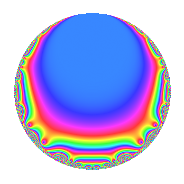# Properties

 Label 6003.2.a.fLevel 6003 Weight 2 Character orbit 6003.a Self dual Yes Analytic conductor 47.934 Analytic rank 1 Dimension 2 CM No Inner twists 1

# Related objects

## Newspace parameters

 Level: $$N$$ = $$6003 = 3^{2} \cdot 23 \cdot 29$$ Weight: $$k$$ = $$2$$ Character orbit: $$[\chi]$$ = 6003.a (trivial)

## Newform invariants

 Self dual: Yes Analytic conductor: $$47.9341963334$$ Analytic rank: $$1$$ Dimension: $$2$$ Coefficient field: $$\Q(\sqrt{5})$$ Coefficient ring: $$\Z[a_1, \ldots, a_{11}]$$ Coefficient ring index: $$2^{2}$$ Fricke sign: $$1$$ Sato-Tate group: $\mathrm{SU}(2)$

## $q$-expansion

Coefficients of the $$q$$-expansion are expressed in terms of $$\beta = \frac{1}{2}(1 + \sqrt{5})$$. We also show the integral $$q$$-expansion of the trace form.

 $$f(q)$$ $$=$$ $$q + q^{2} - q^{4} + 2 q^{5} -3 q^{8} +O(q^{10})$$ $$q + q^{2} - q^{4} + 2 q^{5} -3 q^{8} + 2 q^{10} + ( 4 - 4 \beta ) q^{11} -2 q^{13} - q^{16} + ( -2 + 4 \beta ) q^{17} + ( -4 + 4 \beta ) q^{19} -2 q^{20} + ( 4 - 4 \beta ) q^{22} + q^{23} - q^{25} -2 q^{26} - q^{29} -8 q^{31} + 5 q^{32} + ( -2 + 4 \beta ) q^{34} + ( -2 + 4 \beta ) q^{37} + ( -4 + 4 \beta ) q^{38} -6 q^{40} + ( 6 - 8 \beta ) q^{41} + ( 4 - 4 \beta ) q^{43} + ( -4 + 4 \beta ) q^{44} + q^{46} + ( -8 + 8 \beta ) q^{47} -7 q^{49} - q^{50} + 2 q^{52} + 2 q^{53} + ( 8 - 8 \beta ) q^{55} - q^{58} + 4 q^{59} + ( -10 + 4 \beta ) q^{61} -8 q^{62} + 7 q^{64} -4 q^{65} -4 q^{67} + ( 2 - 4 \beta ) q^{68} -8 q^{71} + ( -6 + 8 \beta ) q^{73} + ( -2 + 4 \beta ) q^{74} + ( 4 - 4 \beta ) q^{76} + ( 8 - 4 \beta ) q^{79} -2 q^{80} + ( 6 - 8 \beta ) q^{82} -4 q^{83} + ( -4 + 8 \beta ) q^{85} + ( 4 - 4 \beta ) q^{86} + ( -12 + 12 \beta ) q^{88} + ( 6 - 12 \beta ) q^{89} - q^{92} + ( -8 + 8 \beta ) q^{94} + ( -8 + 8 \beta ) q^{95} + ( 10 - 12 \beta ) q^{97} -7 q^{98} +O(q^{100})$$ $$\operatorname{Tr}(f)(q)$$ $$=$$ $$2q + 2q^{2} - 2q^{4} + 4q^{5} - 6q^{8} + O(q^{10})$$ $$2q + 2q^{2} - 2q^{4} + 4q^{5} - 6q^{8} + 4q^{10} + 4q^{11} - 4q^{13} - 2q^{16} - 4q^{19} - 4q^{20} + 4q^{22} + 2q^{23} - 2q^{25} - 4q^{26} - 2q^{29} - 16q^{31} + 10q^{32} - 4q^{38} - 12q^{40} + 4q^{41} + 4q^{43} - 4q^{44} + 2q^{46} - 8q^{47} - 14q^{49} - 2q^{50} + 4q^{52} + 4q^{53} + 8q^{55} - 2q^{58} + 8q^{59} - 16q^{61} - 16q^{62} + 14q^{64} - 8q^{65} - 8q^{67} - 16q^{71} - 4q^{73} + 4q^{76} + 12q^{79} - 4q^{80} + 4q^{82} - 8q^{83} + 4q^{86} - 12q^{88} - 2q^{92} - 8q^{94} - 8q^{95} + 8q^{97} - 14q^{98} + O(q^{100})$$

## Embeddings

For each embedding $$\iota_m$$ of the coefficient field, the values $$\iota_m(a_n)$$ are shown below.

For more information on an embedded modular form you can click on its label.

Label $$\iota_m(\nu)$$ $$a_{2}$$ $$a_{3}$$ $$a_{4}$$ $$a_{5}$$ $$a_{6}$$ $$a_{7}$$ $$a_{8}$$ $$a_{9}$$ $$a_{10}$$
1.1
 1.61803 −0.618034
1.00000 0 −1.00000 2.00000 0 0 −3.00000 0 2.00000
1.2 1.00000 0 −1.00000 2.00000 0 0 −3.00000 0 2.00000
 $$n$$: e.g. 2-40 or 990-1000 Significant digits: Format: Complex embeddings Normalized embeddings Satake parameters Satake angles

## Inner twists

This newform does not admit any (nontrivial) inner twists.

## Atkin-Lehner signs

$$p$$ Sign
$$3$$ $$-1$$
$$23$$ $$-1$$
$$29$$ $$1$$

## Hecke kernels

This newform can be constructed as the intersection of the kernels of the following linear operators acting on $$S_{2}^{\mathrm{new}}(\Gamma_0(6003))$$:

 $$T_{2} - 1$$ $$T_{5} - 2$$